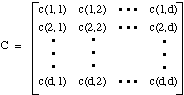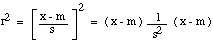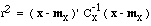# Covariance Matrix

All of the covariances c(i,j) can be collected together into a covariance matrix C:This matrix provides us with a way to measure distance that is invariant to linear transformations of the data. Suppose that we start with a d-dimensional feature vector x that has a mean vector mx and a covariance matrix Cx. If we use the d-by-d matrix A to transform x into y through

y = A x ,

it is not hard to show that the mean vector for y is given by

my = A mx,

and the covariance matrix for y is given by

Cy = A Cx A' .

Suppose now that we want to measure the distance from x to mx, or from y to my. We could, of course, use the Euclidean norm, but it would be very unusual if the Euclidean distance from x to mx turned out to be the same as the Euclidean distance from y to my. (Geometrically, that would happen only if A happened to correspond to a rotation or a reflection, which is not very interesting.) What we want to do is to normalize the distance, much like we did when we defined the standardized distance for a single feature. The question is: What is the matrix generalization of the scalar expressionThe answer turns out to be.

If you know some linear algebra, you should be able to prove that this expression is invariant to any nonsingular linear transformation. That is, if you substitute y = A x and use the formulas above for my and Cy, you will get the very same numerical value for r, no matter what the matrix A is.*

Now, suppose there is a feature space in which the clusters are spherical and the Euclidean metric provides the right way to measure the distance from y to my. In that space, the covariance matrix is the identity matrix, and r is exactly the Euclidean distance from y to my. But since we can get to that space from the x space through a linear transformation, and since r is invariant to linear transformation, we can equally well compute r directly from.